Next: B. Device Model Implementation Up: Dissertation Grasser Previous: 8. Outlook

# A. Thermal EquivalentsSolving the one-dimensional static lattice heat flow equation

 div SL = H -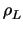. cL .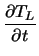(A1) SL = -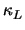. grad TL (A2)

one can derive a thermal equivalent model for a device with a geometry as shown in Fig. A.1a. With the boundary conditions TL(- a) = T1 and TL(a) = T2 and under the assumption of constant heat generation H the solution for the lattice temperature inside the device reads

 TL(x) =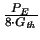.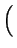1 -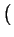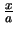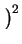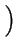-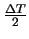.+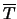(A3)

with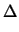T = T1 - T2,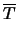=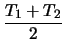(A4) PE = H . A . 2 . a, Gth =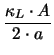. (A5)

It consists of a linear term arising from the boundary condition and a quadratic term arising from heat generation inside the device due to the dissipated electrical power PE. The temperature distribution is shown in Fig. A.1b.

To derive a thermal equivalent circuit the discretized lattice heat flow equation as solved by MINIMOS-NT can be used. Assuming constant electrical power dissipation PE and a constant thermal heat capacity cL the expression for a grid point i reads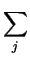Pi, j =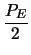+ Cth .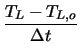(A6) Pi, j = Gth .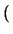Ti - Tj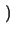(A7) Cth = Vi .. cL   . (A8)

The sum in (A.6) considers the contribution of all neighbor points j with Pi, j being the thermal heat flowing from point i to point j. Using heat flows instead of electrical currents and temperatures instead of voltages an electrical equivalent circuit can be used to model (A.6). This equivalent circuit is shown in Fig. A.2 for grid point i in a two-dimensional situation with four neighbor points.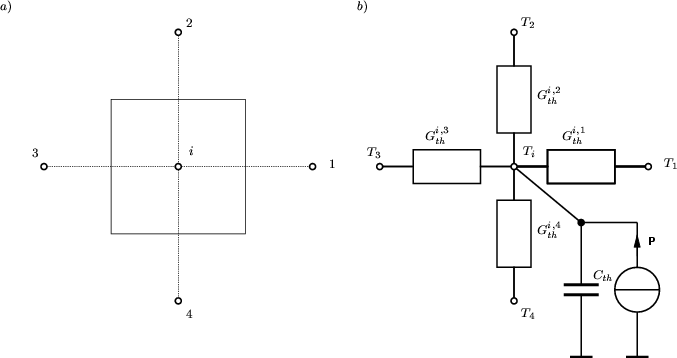This equivalent circuit is approximately valid for the most general case. The geometry of a schematic layout is shown in Fig. A.3. To keep the problem tractable, device simulation is normally restricted to the electrical active regions which make up only a small portion of the chip. Heat generation is even further restricted to small areas of the device which normally are the space charge regions for bipolar devices and the channel regions for MOS devices. For other areas like buried collectors and substrates heat generation is normally negligible as either the electric field, the current density, or both are low. Therefore the heat generation term H can be neglected for these areas and the thermal equivalent circuit reduces to resistances and capacitances.

On the other hand, for the electrical active region the thermal resistances and capacitances are of minor importance due to their small size. This is the reason why power dissipation in electrical devices is normally modeled by a power source alone which can be considered as reducing the power source to a point source.

It is worthwhile to point out the simplifying assumptions made in the derivations above:

• A constant thermal conductivity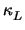has been assumed which is a very crude approximation. Using the exponential expression from= 1.5486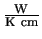.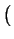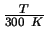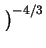(A9)

gives and error in the solution of the linear heat diffusion equation in the order of 30 % .
• Heat generation has been assumed constant inside the device. This gives a good approximation only when the temperature rise due to H is small compared to the temperature rise induced by the boundary condition.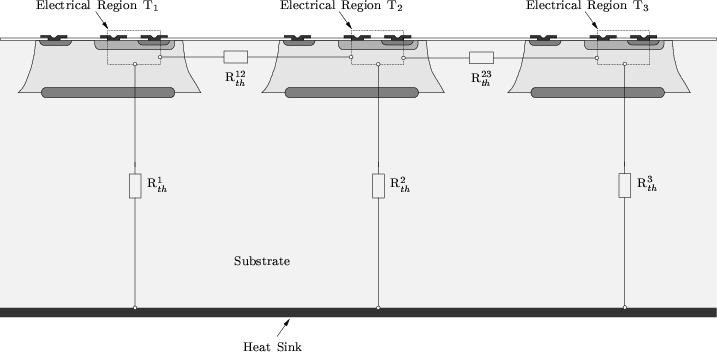Next: B. Device Model Implementation Up: Dissertation Grasser Previous: 8. Outlook
Tibor Grasser
1999-05-31